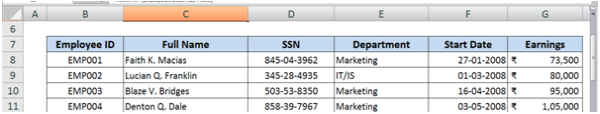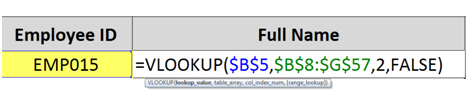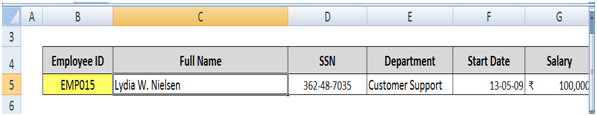# Excellence in Excel - Part III

How to use VLOOKUP in Excel

On many request to write an article on VLOOKUP,I tried my best to fulfill the requests.

VLOOKUP is a powerful Excel function that allows you to look for a specified value in one column of data inside a table, and then fetch a value from another column in the same row.

An example where VLOOKUP might be useful is if you have an employee salary report in Excel, and want to find the name or department or starting date or salary of a specific employee identified by unique ID from within a salary report. You would look up the Employee’s ID in the ID column, and then look in the Name/department/starting date/salary column to find that employee's details.

VLOOKUP() Function Syntax

The VLOOKUP() function has the following syntax:

= VLOOKUP(lookup_value, table_array, col_index_num, [range lookup])

VLOOKUP() works by taking the value you are looking up (lookup_value) and looking for it in the first column of the table you are searching in (table_array).

Once the function finds the matching value in the table_array, it then reads across that row the table to the column you chose and returns the value it finds there.

In some cases you want the VLOOKUP function to find the nearest match, and sometimes you'll want the exact match. In this function, range lookup is an optional value (that's why it's not shown in bold above).If you set the range lookup  parameter to 1, the formula will look for the nearest value (approximate value). If you set it to 0 or FALSE (or leave it out), it will look for an exact match and return a #N/A error if it can't find an exact match.

NOTE: Approximate value i.e. 1 is set as range lookup value in case of slabs like income tax slabs. If data table is not in the form of slab, use exact match option or leave it out.

Examples of the VLOOKUP() function in action - VLOOKUP with an exact match

Here is an example of how the VLOOKUP() function is used to find a value based on an exact match.

Suppose you have a table that looks like the following:- You've been asked to come up with a way to display the name, department, Starting date and salary of an employee when an employee ID is typed into a given cell (B5).

- The formula you type in looks like this:- In the table above, the VLOOKUP function looks for the value found in B5 (EMP015) in the first column of the range of cells from B7:G57 (i.e. the table in the previous step).

- Having found it in the first column of the selected table array (column B) it then reads across to the second column i.e. Column no 2 (column C) and retrieves the value. In this case, the value retrieved is “Lydia W. Nielsen”.

- Copy the formula to other cells to retrieve SSN, Department, Start Date and salary for specified Employee ID.i. In case you're wondering, the \$ signs in the function (\$B\$7:\$G\$57) mean that if you copy the formula in C5 to another location, it will continue to look in the employee table. This is an example of absolute references in action.

ii. Note that if your VLOOKUP function generates a #N/A error, it could be because you didn't use absolute references, and then copied and pasted the VLOOKUP formula from one cell to another

iii. No doubt you must have spotted the FALSE as the fourth parameter in the formula in C5. This means that the VLOOKUP function is looking for an exact match. If it hadn't found the value from B5 (EMP015) in the first column of the table, the formula in C5 would have returned a #N/A error, meaning Excel couldn't return a value from the function. Remember, of course, that the exact parameter is optional, and defaults to 0 if you don't include it, so in this case we could have left this value out altogether and got the same result.

iv. If we had put 1 instead of 0 in our formula in the example above, the VLOOKUP function would have looked down the list in column B until either it found the value it was looking for, or it found a value that was larger than the value it was looking for, and then taken the previous value in column B as the nearest match.

v. Column Number to be mentioned in the VLOOKUP formula is counted as per the column in the table not from the excel spreadsheet.

vi. Rememeber: Value to be looked upon must be contained in the first column of the table array, not in any other column.

vii. It's worth noting that it is very common when using the VLOOKUP table to get the design of the lookup table wrong. If your VLOOKUP function isn't working properly, check the design of the table first to make sure that it is correct.

As I said earlier, the VLOOKUP() function in Excel is very powerful. These are two examples of how to use it, but there are many more ways it can be applied. If you have a scenario where you think the VLOOKUP() function might apply but you can't figure it out, tell me about it in the comments below.

I hope you all liked the article. Feel free to comment and give suggestions for improvements.

Use Thank User option if you find the article helpful

Tags :

Category Info Technology
Other Articles by -
CA ANKUSH AGGARWAL

Report Abuse

### Popular Articles#### Extension of Income Tax and GST Due Dates amid Second Wave of COVID-19 - Need of the hour?#### TDS deducted? Be careful if you are filing ITR only with Form 26AS#### New Tax Regime#### Tax Compliance and Statutory due dates for the m/o May, 2021#### Relief Measures declared by CBIC and CBDT in Indirect Tax and Direct Tax#### Tax Planning tips for individuals & HUF for FY 2021-22#### Covid-19 Relief Measures for Taxpayers under GST law#### What does getting the prefix CA feels like?

CCI Articles